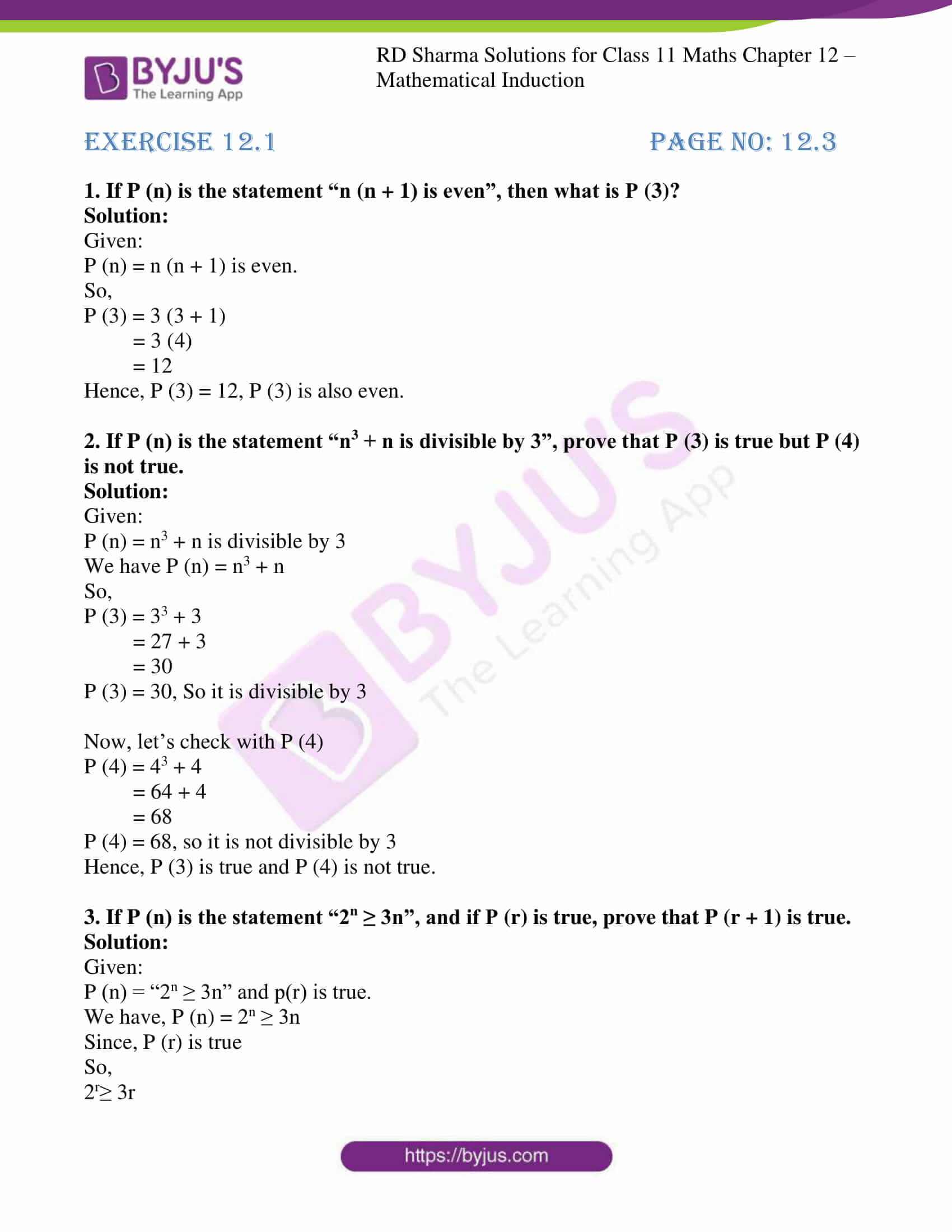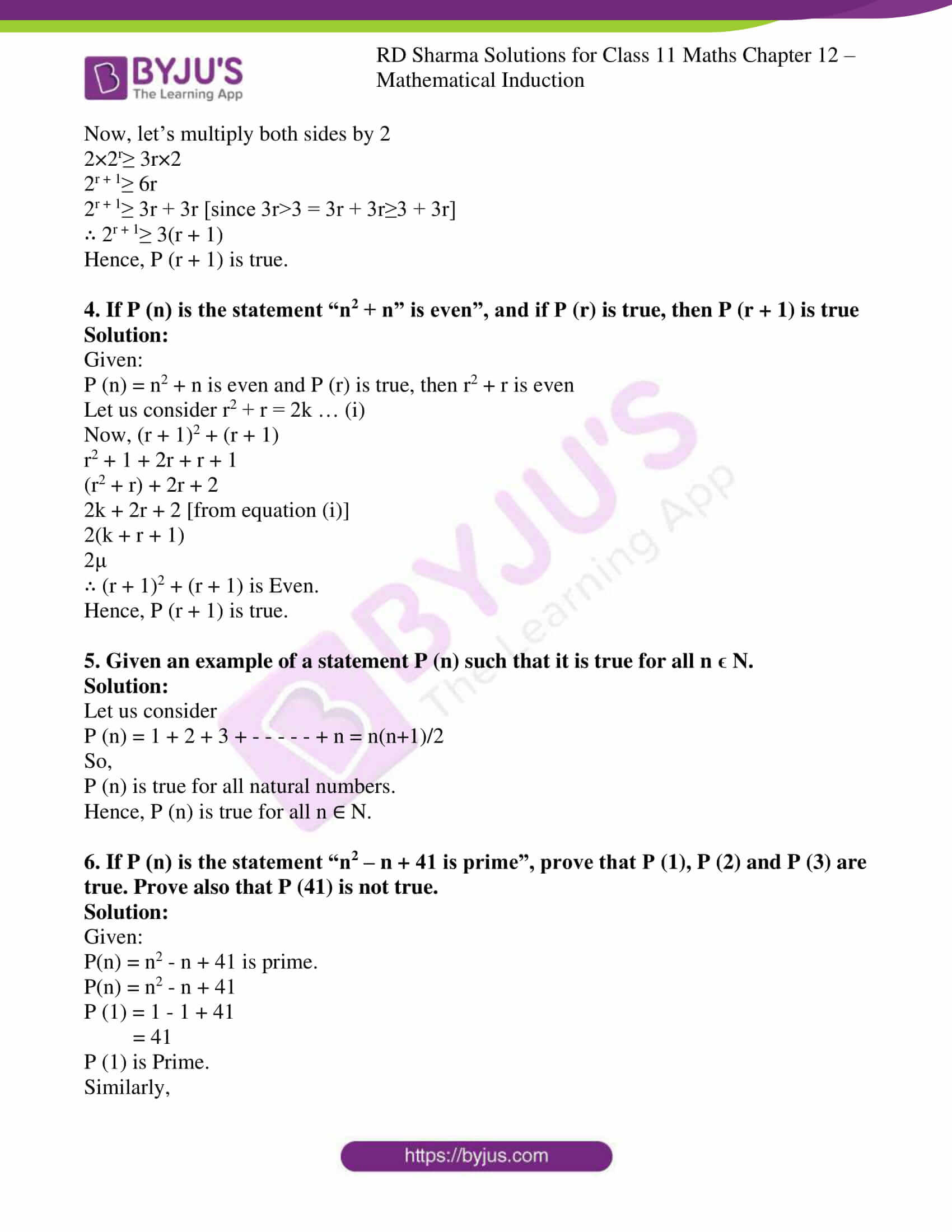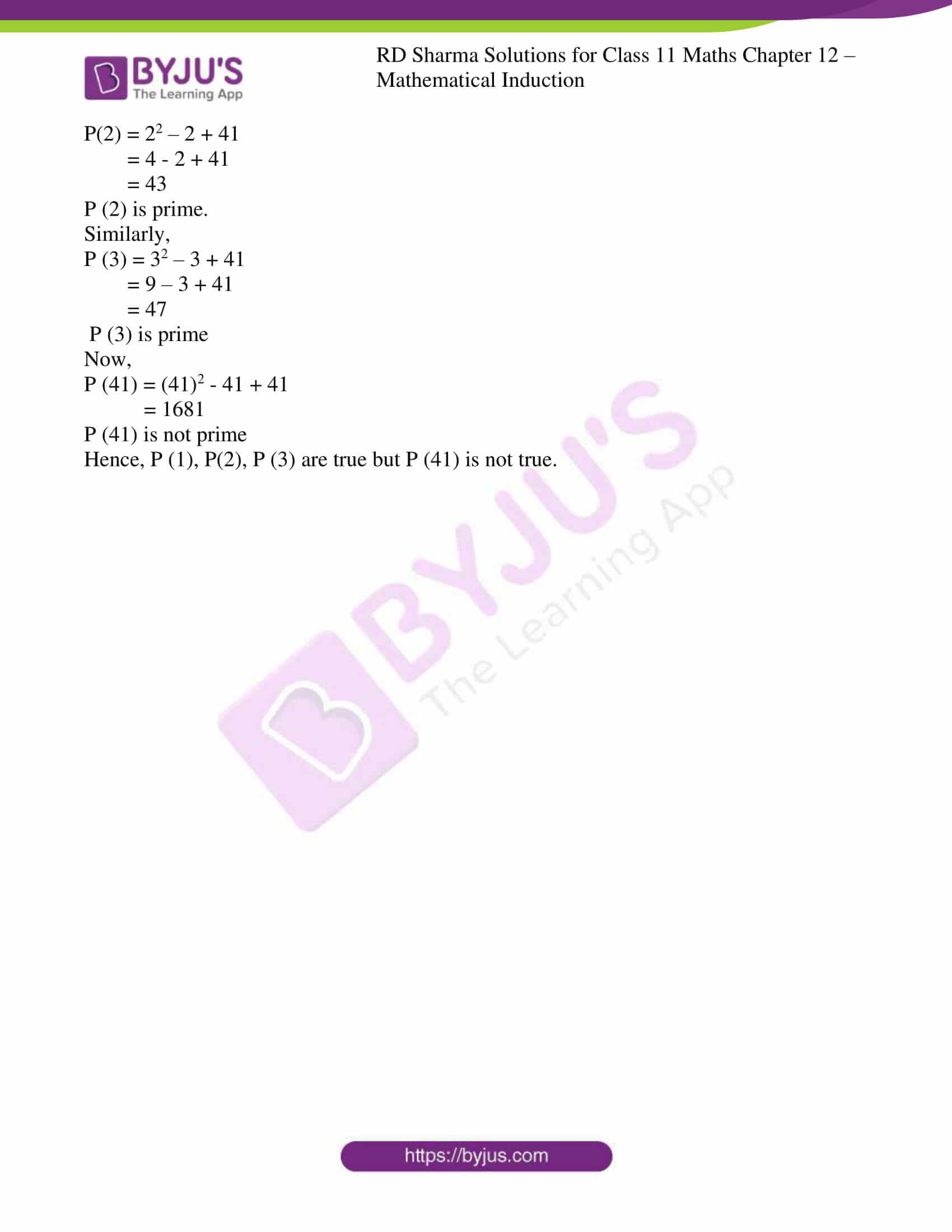# RD Sharma Solutions for Class 11 Chapter 12 - Mathematical Induction Exercise 12.1

In Exercise 12.1 of Chapter 12, we shall discuss problems based on the first principle of mathematical induction. Students can refer to RD Sharma Class 11 Maths as the reference material to score high marks and come out with flying colours in their board exams. Keeping in mind the concepts, subject experts have prepared the solutions in accordance with the latest CBSE syllabus. The solutions are solved in the step by step format using shortcut methods where every student can easily remember the steps. Students can easily download the RD Sharma Class 11 Solutions for Maths, which is available in the pdf format from the links given below.

## Download the Pdf of RD Sharma Solutions for Class 11 Maths Exercise 12.1 Chapter 12 – Mathematical Induction### Access answers to RD Sharma Solutions for Class 11 Maths Exercise 12.1 Chapter 12 – Mathematical Induction

1. If P (n) is the statement “n (n + 1) is even”, then what is P (3)?
Solution:

Given:

P (n) = n (n + 1) is even.

So,

P (3) = 3 (3 + 1)

= 3 (4)

= 12

Hence, P (3) = 12, P (3) is also even.

2. If P (n) is the statement “n3 + n is divisible by 3”, prove that P (3) is true but P (4) is not true.

Solution:

Given:

P (n) = n3 + n is divisible by 3

We have P (n) = n3 + n

So,

P (3) = 33 + 3

= 27 + 3

= 30

P (3) = 30, So it is divisible by 3

Now, let’s check with P (4)

P (4) = 43 + 4

= 64 + 4

= 68

P (4) = 68, so it is not divisible by 3

Hence, P (3) is true and P (4) is not true.

3. If P (n) is the statement “2n ≥ 3n”, and if P (r) is true, prove that P (r + 1) is true.

Solution:

Given:

P (n) = “2n ≥ 3n” and p(r) is true.

We have, P (n) = 2n ≥ 3n

Since, P (r) is true

So,

2r≥ 3r

Now, let’s multiply both sides by 2

2×2r≥ 3r×2

2r + 1≥ 6r

2r + 1≥ 3r + 3r [since 3r>3 = 3r + 3r≥3 + 3r]

∴ 2r + 1≥ 3(r + 1)

Hence, P (r + 1) is true.

4. If P (n) is the statement “n2 + n” is even”, and if P (r) is true, then P (r + 1) is true
Solution:

Given:

P (n) = n2 + n is even and P (r) is true, then r2 + r is even

Let us consider r2 + r = 2k … (i)

Now, (r + 1)2 + (r + 1)

r2 + 1 + 2r + r + 1

(r2 + r) + 2r + 2

2k + 2r + 2 [from equation (i)]

2(k + r + 1)

∴ (r + 1)2 + (r + 1) is Even.

Hence, P (r + 1) is true.

5. Given an example of a statement P (n) such that it is true for all n ϵ N.

Solution:

Let us consider

P (n) = 1 + 2 + 3 + – – – – – + n = n(n+1)/2

So,

P (n) is true for all natural numbers.

Hence, P (n) is true for all n ∈ N.

6. If P (n) is the statement “n2 – n + 41 is prime”, prove that P (1), P (2) and P (3) are true. Prove also that P (41) is not true.
Solution:

Given:

P(n) = n2 – n + 41 is prime.

P(n) = n2 – n + 41

P (1) = 1 – 1 + 41

= 41

P (1) is Prime.

Similarly,

P(2) = 22 – 2 + 41

= 4 – 2 + 41

= 43

P (2) is prime.

Similarly,

P (3) = 32 – 3 + 41

= 9 – 3 + 41

= 47

P (3) is prime

Now,

P (41) = (41)2 – 41 + 41

= 1681

P (41) is not prime

Hence, P (1), P(2), P (3) are true but P (41) is not true.